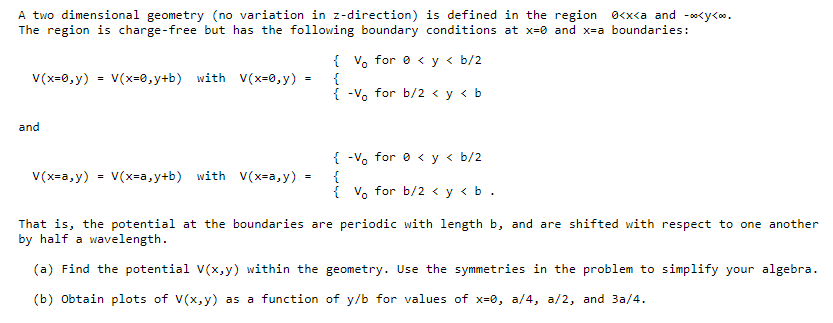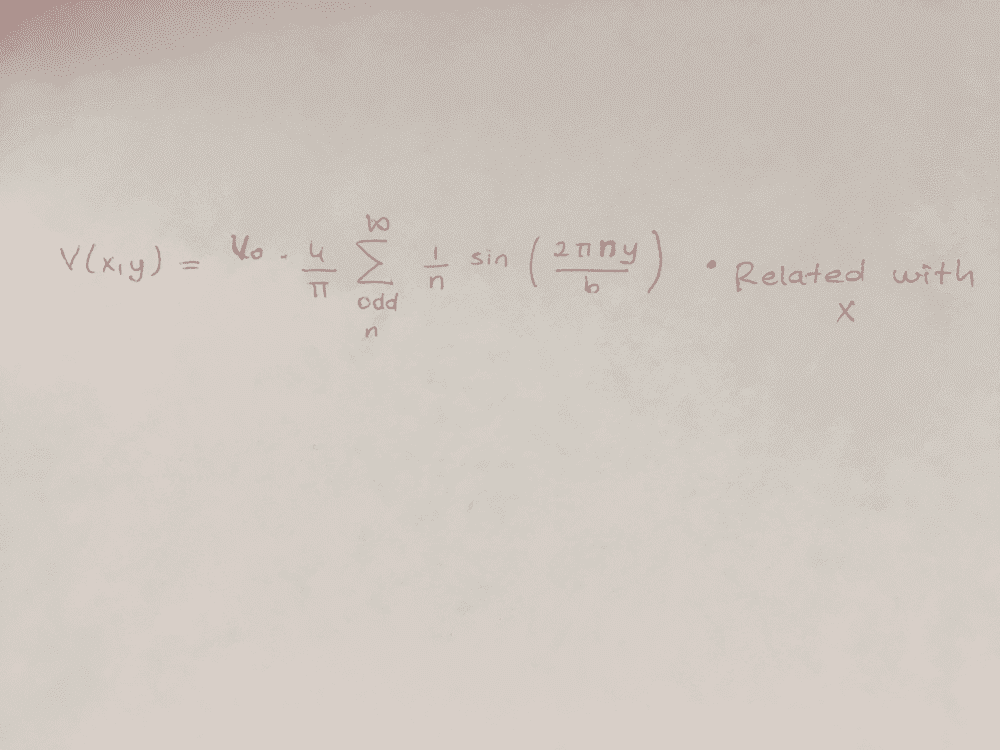# Boundary Potential Problem- parallel alternating potential

ebru
Homework Statement:
Hello,
I have a question about the potential. I think the potential shoul be something like below. In that way periodic behavior work perfectly. But I don't know how to add the x variable to the equation.
Relevant Equations:
So how can I find potential?#### Attachments

$$\Delta \phi = -\frac{\rho}{\epsilon} = 0$$